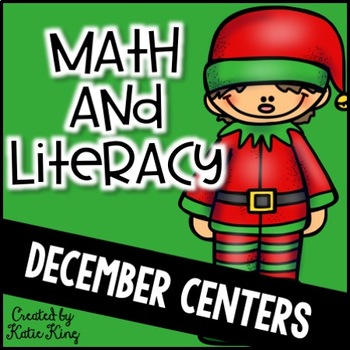# All I want for Christmas: 10 Common Core Literacy Centers AND 10 Math CentersSubject
Resource Type
Product Rating
File Type

PDF (Acrobat) Document File

38 MB|160 pages
Share
Product Description
Reindeer Line-Up! ABC Order: R. FS.3G.-Recognize and read grade-appropriate irregularly spelled words.

Santa’s Sleigh of Sentences: R.FS.1.A- Recognize the distinguishing features of a sentence (e.g., first word,
capitalization, ending punctuation).

North Pole Nouns- Proper vs. Common Nouns: LS.1B.- Use common, proper, and possessive nouns

Digraph Dash: R.FS.3.A.-Know the spelling-sound correspondences for common consonant digraphs

Stocking Syllable Sort: R.FS.3D.- Use knowledge that every syllable must have a vowel sound to determine the number of syllables in a printed word.
Christmas Contractions: R.L.2C.- Use an apostrophe to form contractions and frequently occurring possessives.

Trimming Trees with Long and Short Vowels: R.FS.3C. -Know final -e and common vowel team conventions for representing long vowel sounds.

Describe Me: R.L.4.- Identify words and phrases in stories or poems
that suggest feelings or appeal to the senses.

Word Family Trees: R.FS.1.B. -Orally produce single-syllable words by blending sounds (phonemes),
including consonant blends.

Stocking Inflectional Endings: R. FS.3.F.- Read words with inflectional endings.
Place Value Presents: 1.M. NBT2.-Understand that the two digits of a two-digit number represent amounts of tens and ones.

Let it Snow- A Game of Time: 1.M. MD.3.-Tell and write time in hours and half-hours using analog and digital clocks.

Elfie Learns to Measure: 1.M. MD.2.-Express the length of an object as a whole number of length units, by laying multiple copies of a shorter object (the length unit) end to end;
understand that the length measurement of an object is the number of same-size length units that span it with no gaps or overlaps. Limit to
contexts where the object being measured is spanned by a whole number of length units with no gaps or overlaps.

Stocking Subtraction Match: 1.M.OA.6.-Add and subtract within 20, demonstrating fluency for addition and subtraction within 10.

Fill Up Santa’s Bag: 1.M.OA.1-Use addition and subtraction within 20 to solve word problems involving situations of adding to, taking from, putting together, taking apart, and comparing, with unknowns in all positions, e.g., by using objects, drawings, and equations with a symbol for the unknown number to represent the problem.
Santa’s on Top of the Roof: 1. M.NBT.3-Compare two two-digit numbers based on meanings of the tens and ones digits, recording the results of comparisons with the symbols >, =, and <.

Fa-la-lah Fact Famlies: 1.M.OA.3- Apply properties of operations as strategies to add and subtract.3 Examples: If 8 + 3 = 11 is known, then 3 + 8 = 11 is also known. (Commutative property of addition.) To add 2 + 6 + 4, the second two numbers can be added to make a ten, so 2 + 6 + 4 = 2 + 10 = 12. (Associative property of addition.)

Missing Link in Rudolph’s Crew: 1.M.OA.8- Determine the unknown whole number in an addition or subtraction equation relating three whole numbers. For example, determine the unknown number that makes the equation true in each of the equations 8 + ? = 11, 5 = ? – 3, 6 + 6 = ?.

Adding up those Sweets: 1.M.OA.2.-Solve word problems that call for addition of three whole numbers whose sum is less than or equal to 20, e.g., by using objects, drawings, and equations with a symbol for the unknown number to represent the problem.
Kickin’ Up 10 More/10 Less: 1.M..NBT.4-. Add within 100, including adding a two-digit number and a one-digit
number, and adding a two-digit number and a multiple of 10
Total Pages
160 pages
N/A
Teaching Duration
N/A
Report this Resource to TpT
Reported resources will be reviewed by our team. Report this resource to let us know if this resource violates TpT’s content guidelines.